# 基于天空区域分割的单幅图像去雾方法Single Image Dehazing Method Based on Sky Region Segmentation

DOI: 10.12677/CSA.2020.102033, PDF, HTML, XML, 下载: 272  浏览: 683

Abstract: For the dark channel, a priori is not suitable for the sky area, resulting in a small transmittance and darkening the color of the restored fog-free image. Based on the segmentation of the sky re-gion, it is proposed to separately estimate the atmospheric intensity defogging process according to different regions. Improved threshold segmentation method makes it more accurate to distinguish sky domain from non-sky domain. The white edge generated after segmentation is processed by the fusion method, which reduces the white edge effect at the boundary and makes the picture more natural after defogging. The experimental results show that the segmentation speed is fast, can achieve a good defogging effect and has no obvious color patches for the sky area, and the image is more natural and clear after defogging.

1. 引言

2. 基于暗原色先验模型

2.1. 大气散射函数

${E}_{D}\left(d,\lambda \right)={E}_{0}\left(\lambda \right){\text{e}}^{-\beta \left(\lambda \right)d}$ (式1-1)

${E}_{A}\left(d,\lambda \right)={E}_{w}\left(\lambda \right)\left(1-{\text{e}}^{-\beta \left(\lambda \right)d}\right)$ (式1-2)

$E\left(d,\lambda \right)={E}_{D}\left(d,\lambda \right)+{E}_{A}\left(d,\lambda \right)$ (式1-5)

$E\left(d,\lambda \right)={E}_{0}\left(\lambda \right){\text{e}}^{-\beta \left(\lambda \right)d}+{E}_{w}\left(\lambda \right)\left(1-{\text{e}}^{-\beta \left(\lambda \right)d}\right)$ (式1-6)

$I\left(x\right)=E\left(d,\lambda \right)$$t\left(x\right)={\text{e}}^{-\beta \left(\lambda \right)d}$$J\left(x\right)={E}_{0}\left(\lambda \right)$$A={E}_{w}\left(\lambda \right)$ 式得

$I\left(x\right)=J\left(x\right)t\left(x\right)+A\left(1-t\left(x\right)\right)$ (式1-7)

2.2. 暗原色先验原理

He等  基于对无雾图像的大量统计发现对于绝大多数非天空区域，至少存在一个颜色通道值很低的像素，且接近于0。提出暗通道先验模型，对于任何一个无雾图像J可以表示为：

${J}^{dark}\left(x\right)=\underset{y\in \Omega \left(x\right)}{\mathrm{min}}\left(\underset{c\in \left\{r,g,b\right\}}{\mathrm{min}}\left({J}^{c}\left(y\right)\right)\right)$ (式1-8)

$\frac{{I}^{C}\left(x\right)}{{A}^{C}}=\stackrel{˜}{t}\left(x\right)\frac{{J}^{C}\left(x\right)}{{A}^{C}}+1-\stackrel{˜}{t}\left(x\right)$ (式1-9)

$\underset{y\in \Omega \left(x\right)}{\mathrm{min}}\left(\underset{c}{\mathrm{min}}\left(\frac{{I}^{C}\left(y\right)}{{A}^{C}}\right)\right)=\stackrel{˜}{t}\left(x\right)\underset{y\in \Omega \left(x\right)}{\mathrm{min}}\left(\underset{c}{\mathrm{min}}\left(\frac{{J}^{C}\left(y\right)}{{A}^{C}}\right)\right)+1-\stackrel{˜}{t}\left(x\right)$ (式1-10)

$\stackrel{˜}{t}\left(x\right)=\frac{1-\underset{y\in \Omega \left(x\right)}{\mathrm{min}}\left(\underset{c}{\mathrm{min}}\left(\frac{{I}^{C}\left(y\right)}{{A}^{C}}\right)\right)}{1-\underset{y\in \Omega \left(x\right)}{\mathrm{min}}\left(\underset{c}{\mathrm{min}}\left(\frac{{J}^{C}\left(y\right)}{{A}^{C}}\right)\right)}$ (式1-11)

${J}^{dark}\left(x\right)=\underset{y\in \Omega \left(x\right)}{\mathrm{min}}\left(\underset{c\in \left\{r,g,b\right\}}{\mathrm{min}}\left({J}^{c}\left(y\right)\right)\right)=0$

$\stackrel{˜}{t}\left(x\right)=1-w\underset{y\in \Omega \left(x\right)}{\mathrm{min}}\left(\underset{c}{\mathrm{min}}\left(\frac{{I}^{C}\left(y\right)}{{A}^{C}}\right)\right)$ (式1-12)

$J\left(x\right)=\frac{I\left(x\right)-A}{\stackrel{˜}{t}\left(x\right)}+A$ (式1-13)

$J\left(x\right)=\frac{I\left(x\right)-A}{\mathrm{max}\left(\stackrel{˜}{t}\left(x\right),{t}_{0}\right)}+A$(式1-14)

3. 改进的去雾方法

3.1. 透射率的获取

${q}_{i}={\sum }_{j}{w}_{ij}\left(I\right){p}_{j}$ (式2-1)

${q}_{k}={a}_{k}{I}_{i}+{b}_{k},\forall i\in {w}_{k}$ (式2-2)

$\left({a}_{k},{b}_{k}\right)$ 是一对线性系数，若输入输出图像的差异最低则满足：

$E\left({a}_{k},{b}_{k}\right)=\underset{i\in {w}_{k}}{\sum }\left({\left({a}_{k}{I}_{i}+{b}_{k}-{p}_{i}\right)}^{2}+\epsilon {a}_{k}^{2}\right)$ (式2-3)

$\left\{\begin{array}{l}{a}_{k}=\frac{\frac{1}{|w|}\underset{i\in {w}_{k}}{\sum }{I}_{i}{p}_{i}-{\mu }_{k}\stackrel{¯}{{p}_{k}}}{{\sigma }_{k}^{2}+\epsilon }\\ {b}_{k}=\stackrel{¯}{{p}_{k}}-{a}_{k}{\mu }_{k}\end{array}$

$\left\{\begin{array}{l}{q}_{i}=\frac{1}{|w|}\underset{i\in {w}_{k}}{\sum }{a}_{k}{I}_{i}+{b}_{k}\\ {q}_{i}=\stackrel{¯}{{a}_{i}}{I}_{i}+\stackrel{¯}{{b}_{i}}\\ \stackrel{¯}{{a}_{i}}=\frac{1}{|w|}\underset{i\in {w}_{k}}{\sum }{a}_{k}\\ \stackrel{¯}{{b}_{i}}=\frac{1}{|w|}\underset{i\in {w}_{k}}{\sum }{b}_{k}\end{array}$ (式2-5)

${W}_{ij}\left({J}^{g}\right)=\frac{1}{{|w|}^{2}}\underset{k:\left(i,j\right)\in {w}_{k}}{\sum }\left(1+\frac{\left({J}_{i}^{g}-{\mu }_{k}\right)\left({J}_{j}^{g}-{\mu }_{k}\right)}{{\sigma }_{k}^{2}+\epsilon }\right)$ (式2-6)

3.2. 获取最优分割阈值及改进

$N={n}_{1}+{n}_{2}+{n}_{3}+\cdots +{n}_{L}$。同时灰度级为i在图中出现的概率，则满足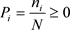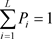。按照阈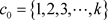,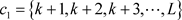(式2-7)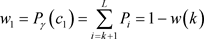(式2-8)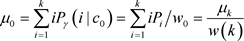(式2-9)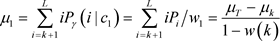(式2-10)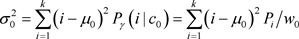(式2-11)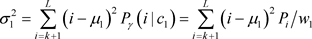(式2-12)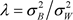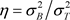。分别表示总体，类内，类间方差。即：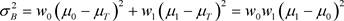(式2-12)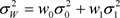(式2-13)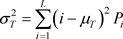(式2-14)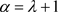(式2-15)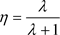(式2-16)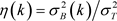(式2-18)(式2-19)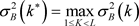(式2-20)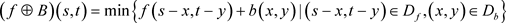(式2-21)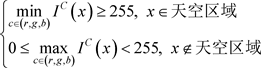(式2-22)

3.3. 大气强度A的获取

1. 非天空区域：统计非天空区域最大亮度的1%像素的平均值为大气光强度A的值 。

2. 天空区域：将有雾图像按上述分割后天空区域均匀分成四块区域，计算平均值和标准值的最大差值与设定的阈值比较，大于阈值的继续均分成四块计算平均值和标准值的差值，当小于设定阈值时，计算选定区域的平均亮度值作为大气光强度的A值。

3.4. 优化白边效应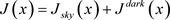(式2-23)

4. 试验结果与分析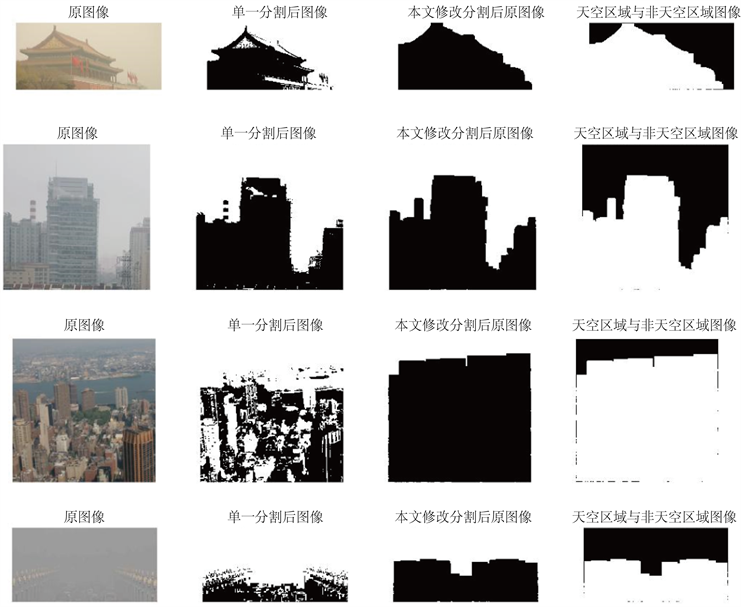Figure 1. Single threshold segmentation and improved threshold segmentationFigure 2. Original transmittance and improved transmittance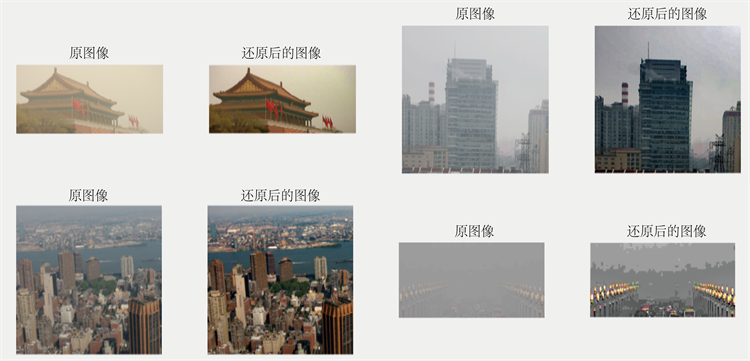Figure 3. Original image and image after defogging

5. 结语

  Kim, T.K., Paik, J.K. and Kang, B.S. (1998) Contrast Enhancement System Using Spatially Adaptive Histogram Equalization with Temporal Filtering. IEEE Transactions on Consumer Electronics, 44, 82-87. https://doi.org/10.1109/30.663733  Xu, H., Guo, J., Liu, Q., et al. (2012) Fast Image Dehazing Using Im-proved Dark Channel Prior. 2012 International Conference on Information Science and Technology (ICIST), Hubei, 23-25 March 2012, 663-667. https://doi.org/10.1109/ICIST.2012.6221729  Ullah, E., Nawaz, R. and Iqbal, J. (2013) Single Image Haze Removal Using Improved Dark Channel Prior. 2013 Proceedings of International Conference on Modelling, Identification & Control (ICMIC), Cairo, 31 August-2 September 2013, 245-248.  Zhu, Q., Mai, J. and Shao, L. (2015) A Fast Single Image Haze Removal Algorithm Using Color Attenuation Prior. IEEE Transactions on Image Processing, 24, 3522-3533. https://doi.org/10.1109/TIP.2015.2446191  Tan, R.T. (2008) Visibility in Bad Weather from a Single Image. CVPR 2008: Proceedings of the 2008 IEEE Conference on Computer Vision and Pattern Recognition, Anchorage, AK, 23-28 June 2008, 1-8. https://doi.org/10.1109/CVPR.2008.4587643  Fattal, R. (2008) Single Image Dehazing. ACM Transactions on Graphics (TOG), 27, Article No. 72. https://doi.org/10.1145/1360612.1360671  Yu, J., Xiao, C. and Li, D. (2010) Physics-Based Fast Single Image Fog Removal. 2010 IEEE 10th International Conference on Signal Processing (ICSP), Beijing, 24-28 October 2010, 1048-1052. https://doi.org/10.1109/ICOSP.2010.5655901  He, K.M., Sun, J. and Tang, X.O. (2011) Single Image Haze Removal Using Dark Channel Prior. IEEE Transactions on Pattern Analysis and Machine Intelligence, 33, 2341-2353. https://doi.org/10.1109/TPAMI.2010.168  Scarr, S. and McCartney, K. (1983) How People Make Their Own Environments: A Theory of Genotype Environment Effects. Child Development, 54, 424-435.  Wahl, S.M., Hunt, D.A., Wakefield, L.M., et al. (1987) Transforming Growth Factor Type Beta Induces Monocyte Chemotaxis and Growth Factor Production. Proceedings of the National Academy of Sciences of the United States of America, 84, 5788-5792. https://doi.org/10.1073/pnas.84.16.5788  Narasimhan, S.G. and Nayar, S.K. (2003) Contrast Restoration of Weather Degraded Images. IEEE Transactions on Pattern Analysis and Machine Intelligence, 25, 713-724. https://doi.org/10.1109/TPAMI.2003.1201821  He, K.M., Sun, J. and Tang, X.O. (2012) Guided Image Filtering. IEEE Transactions on Pattern Analysis and Machine Intelligence, 35, 1397-1409. https://doi.org/10.1109/TPAMI.2012.213  Otsu, N. (1975) A Threshold Selection Method from Gray-Level Histograms. Automatica, 11, 23-27.  徐长新, 彭国华. 二维Otsu阈值法的快速算法[J]. 计算机应用, 2012, 32(5): 1258-1260.  徐振轩. 基于天空区域分割的单幅图像去雾方法研究[D]: [硕士学位论文]. 绵阳: 西南科技大学, 2017.  程炜, 汤红忠, 朱玲, 王翔, 李骁, 郭雪峰. 图像去雾中的大气光强度自适应恢复算法研究[J]. 计算技术与自动化, 2017, 36(1): 103-107.